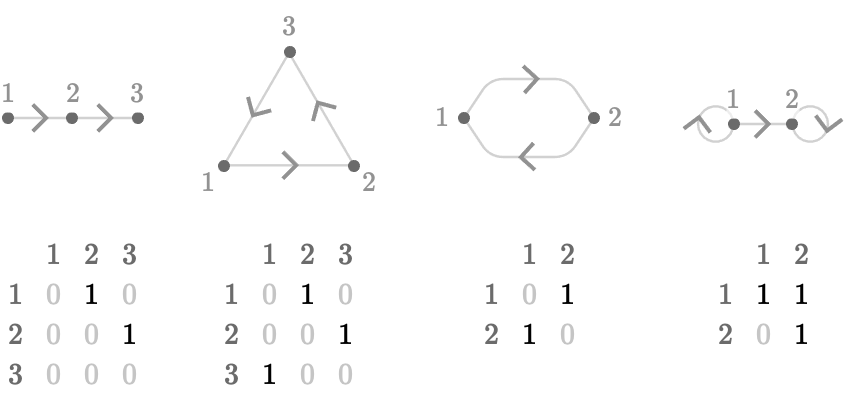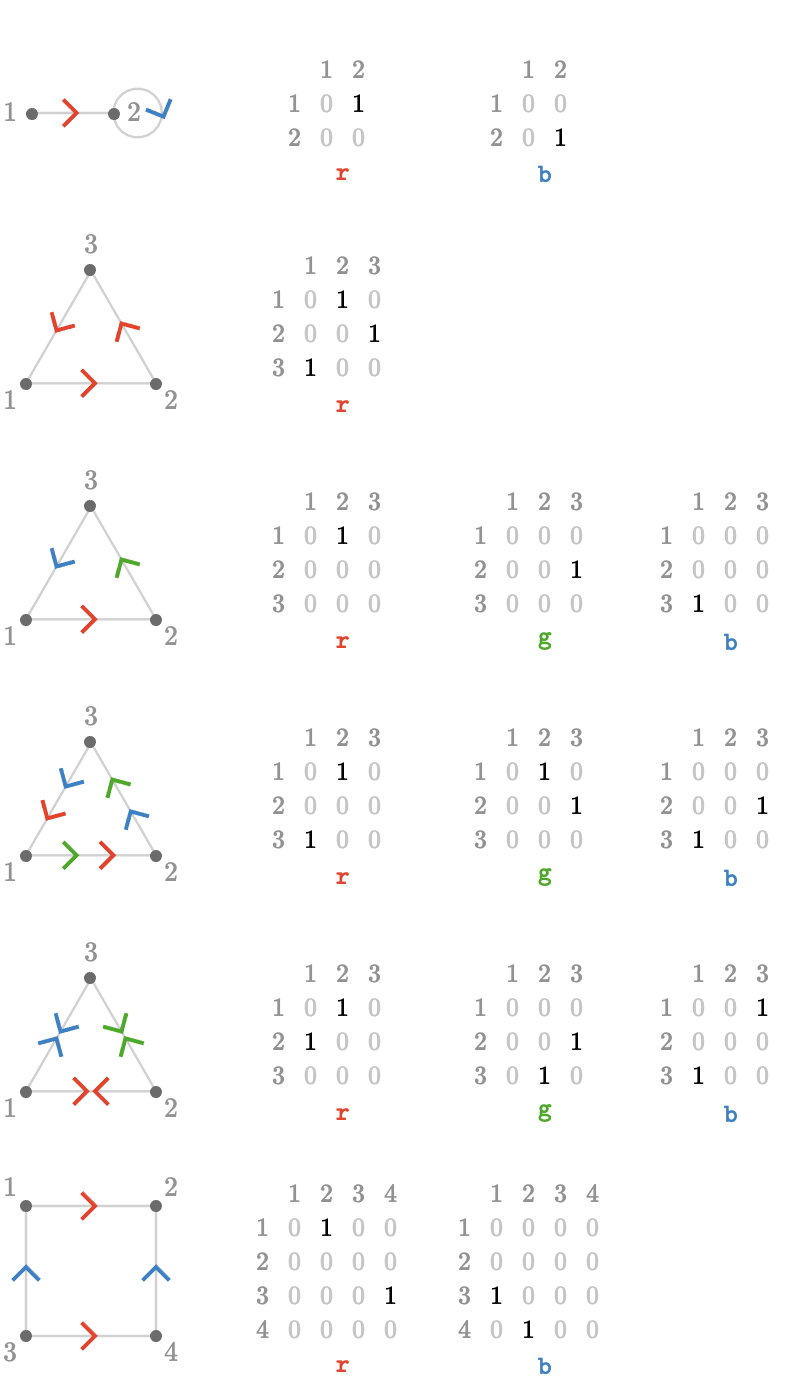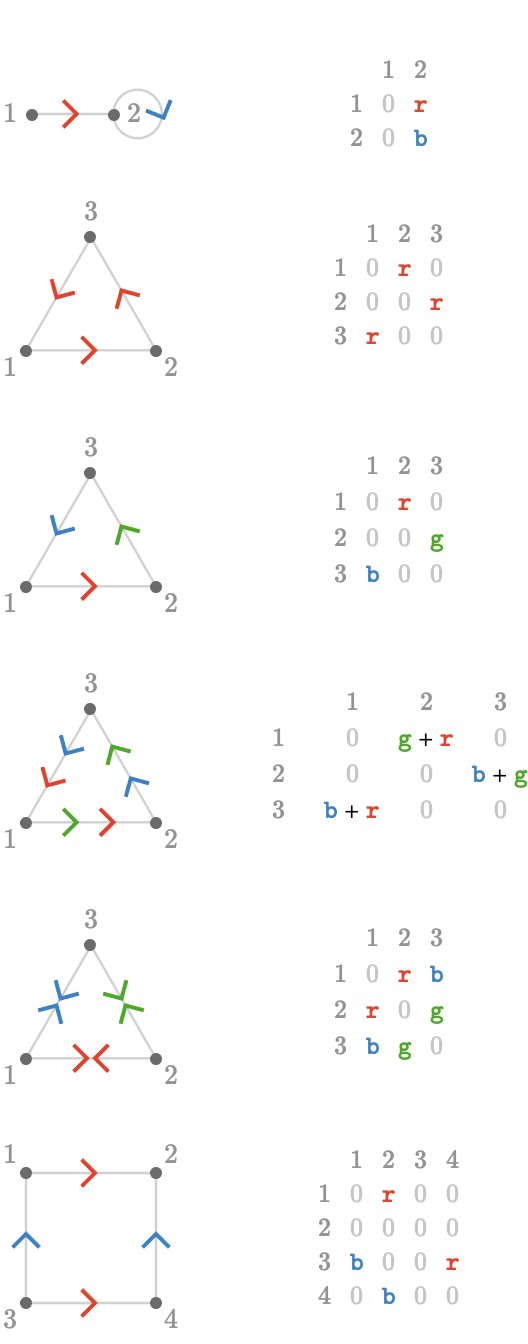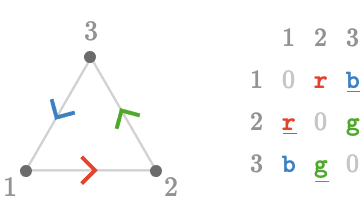$$\gdef\badDispatch#1{\textbf{\textcolor{e1432d}{#1}}} \gdef\noKatexForm#1{\badDispatch{#1}} \gdef\largeDot{\raisebox{0.06em}{\tiny∙}} \gdef\rarrbar{ {\raisebox{-0.05em}{→}\mkern{-0.13em}{\large\shortmid}}} \gdef\larrbar{ { {\large\shortmid}\mkern{-0.13em}{\raisebox{-0.05em}{←}}}} \gdef\suptrans{^\mathsf{T}} \gdef\supdagger{^\dagger} \gdef\rawsymbol#1{\operatorname{#1}} \gdef\colorsymbol#1#2{\textcolor{#1}{\rawsymbol{#2}}} \gdef\lsymbol#1{\colorsymbol{262626}{#1}} \gdef\msymbol#1{\colorsymbol{b50800}{#1}} \gdef\osymbol#1{\colorsymbol{00427f}{#1}} \gdef\lstring#1{\textcolor{666666}{\textrm{\textquotedblleft{\text{#1}}\textquotedblright}}} \gdef\boldForm#1{\textbf{#1}} \gdef\lrform#1{ {\textcolor{e1432d}{#1}}} \gdef\lgform#1{ {\textcolor{4ea82a}{#1}}} \gdef\lbform#1{ {\textcolor{3e81c3}{#1}}} \gdef\lrgform#1{ {\textcolor{ffc87d}{#1}}} \gdef\lgbform#1{ {\textcolor{96e2e3}{#1}}} \gdef\lrbform#1{ {\textcolor{f989b8}{#1}}} \gdef\rform#1{ {\textcolor{cb2b1a}{#1}}} \gdef\gform#1{ {\textcolor{399318}{#1}}} \gdef\bform#1{ {\textcolor{2b6fb0}{#1}}} \gdef\drform#1{ {\textcolor{9b0000}{#1}}} \gdef\dgform#1{ {\textcolor{006800}{#1}}} \gdef\dbform#1{ {\textcolor{004987}{#1}}} \gdef\drgform#1{ {\textcolor{ae5900}{#1}}} \gdef\dgbform#1{ {\textcolor{006567}{#1}}} \gdef\drbform#1{ {\textcolor{86004d}{#1}}} \gdef\rgform#1{ {\textcolor{dc841a}{#1}}} \gdef\gbform#1{ {\textcolor{47a5a7}{#1}}} \gdef\rbform#1{ {\textcolor{c74883}{#1}}} \gdef\pform#1{ {\textcolor{665996}{#1}}} \gdef\dpform#1{ {\textcolor{41326b}{#1}}} \gdef\waform#1{ {\textcolor{6b6b6b}{#1}}} \gdef\wbform#1{ {\textcolor{929292}{#1}}} \gdef\wcform#1{ {\textcolor{c5c5c5}{#1}}} \gdef\tick{\checkmark} \gdef\barToken{\mathbf{|}} \gdef\filledRectangleToken{▮} \gdef\emptyRectangleToken{▯} \gdef\filledSquareToken{■} \gdef\emptySquareToken{□} \gdef\filledToken{∙} \gdef\emptyToken{∘} \gdef\cardinalRewrite#1#2{\rewrite{#1}{#2}} \gdef\primed#1{ {#1}^{\prime}} \gdef\tinybullet{ {\tiny●}} \gdef\factsym#1{\mathord{#1}} \gdef\forwardFactor{\factsym{\uparrow}} \gdef\backwardFactor{\factsym{\downarrow}} \gdef\forwardBackwardFactor{\factsym{\updownarrow}} \gdef\forwardBackwardNeutralFactor{\factsym{\mathrlap{\downarrow}{\mathrlap{\uparrow}{\endash} } }} \gdef\neutralFactor{\factsym{\shornarrow}} \gdef\arrowhead{⏵} \gdef\inverseArrowhead{⏴} \gdef\rightArrowhead{⏵} \gdef\leftArrowhead{⏴} \gdef\upArrowhead{⏶} \gdef\downArrowhead{⏷} \gdef\de#1#2{ { {#1}\xundirectededge{}{#2}}} \gdef\ue#1#2{ { {#1}\xrightedge{}{#2}}} \gdef\shifttag#1{\raisebox{-1em}{#1}} \gdef\shifttag#1{#1} \gdef\tde#1#2#3{ {#1}\:\xrightedge{\shifttag{#3}}\;{#2}} \gdef\tue#1#2#3{ {#1}\;\xundirectededge{\shifttag{#3}}\;{#2}} \gdef\shiftunderset#1#2{\underset{\raisebox{0.15em}{\scriptsize #1}}{#2}} \gdef\mdtde#1#2#3#4{ {#1}\,\;\shiftunderset{#4}{\xrightedge{#3}}\;{#2}} \gdef\mtue#1#2#3#4{ {#1}\,\;\shiftunderset{#4}{\xundirectededge{#3}}\;\,{#2}} \gdef\mtde#1#2#3#4{ {#1}\,\;\operatornamewithlimits{\xrightedge{#3}}\limits_{#4} \;{#2}} \gdef\mapsfrom{\htmlClass{hreflect}{\mapsto}} \gdef\longmapsfrom{\htmlClass{hreflect}{\longmapsto}} \gdef\diffd{𝕕} \gdef\partialdof#1{\partial {#1}} \gdef\emptySet{\empty} \gdef\unknown{\wbform{\text{?}}} \gdef\notApplicable{\wbform{\text{---}}} \gdef\textAnd{\text{\,and\,}} \gdef\identicallyEqualSymbol{\equiv} \gdef\congruentSymbol{\equiv} \gdef\isomorphicSymbol{\simeq} \gdef\homeomorphicSymbol{\cong} \gdef\homotopicSymbol{\simeq} \gdef\approxEqualSymbol{\approx} \gdef\bijectiveSymbol{\approx} \gdef\defeq{\mathrel{≝}} \gdef\defEqualSymbol{\mathrel{≝}} \gdef\syntaxEqualSymbol{\mathrel{\textcolor{888888}{\equiv}}} \gdef\tailEqualSymbol{\underdot{=}} \gdef\headEqualSymbol{\dot{=}} \gdef\ruledelayed{:\to} \gdef\mathdollar{\text{\textdollar}} \gdef\hyphen{\text{-}} \gdef\endash{\text{--}} \gdef\emdash{\text{---}} \gdef\updownarrows{\uparrow\!\downarrow} \gdef\vthinspace{\mkern{1pt}} \gdef\dlq{\text{\textquotedblleft}} \gdef\drq{\text{\textquotedblright}} \gdef\dprime{ {\prime\prime}} \gdef\inverse#1{ { {#1}^{-1}}} \gdef\inverseSymbol{\inverse{□}} \gdef\groupDirectProduct#1{#1} \gdef\groupDirectProductSymbol{\times} \gdef\groupInverse{\inverse} \gdef\groupPower#1#2{ {#1}^{#2}} \gdef\groupCommutator#1#2{\left[{#1},{#2}\right]} \gdef\groupoidInverse{\inverse} \gdef\latticeBFS#1{\textrm{bfs}({#1})} \gdef\pathHomomorphism#1{#1} \gdef\groupoidFunction#1{#1} \gdef\groupoidHomomorphism#1{#1} \gdef\affineModifier#1{\overrightharpoon{#1}} \gdef\supercirc#1{#1^{\circ}} \gdef\supercircb#1{#1^{\bullet}} \gdef\blackCircleModifier#1{\supercircb{#1}} \gdef\whiteCircleModifier#1{\supercirc{#1}} \gdef\totalSpaceStyle#1{\rbform{#1}} \gdef\baseSpaceStyle#1{\bform{#1}} \gdef\fiberSpaceStyle#1{\rform{#1}} \gdef\totalSpaceElementStyle#1{\drbform{#1}} \gdef\baseSpaceElementStyle#1{\dbform{#1}} \gdef\fiberSpaceElementStyle#1{\drform{#1}} \gdef\bundleProjectionStyle#1{\dgform{#1}} \gdef\bundleGraphStyle#1{\rbform{#1}} \gdef\bundleSectionStyle#1{\drgform{#1}} \gdef\bundleFunctionStyle#1{\drbform{#1}} \gdef\graphFunctionStyle#1{\totalSpaceStyle{#1}} \gdef\projectionFunctionStyle#1{\totalSpaceStyle{#1}} \gdef\sectionFunctionStyle#1{\totalSpaceStyle{#1}} \gdef\functionGraph#1{G_{#1}} \gdef\toroidalModifier#1{\supercirc{#1}} \gdef\modulo#1{\supercirc{#1}} \gdef\dividesSymbol{\mathrel{|}} \gdef\groupFunction#1{#1} \gdef\groupHomomorphism#1{#1} \gdef\automorphisms{\operatorname{Aut}} \gdef\endomorphisms{\operatorname{End}} \gdef\transportMap#1{\transportMapSymbol_{#1}} \gdef\transportMapSymbol{\tau} \gdef\action#1{#1} \gdef\selfAction#1{\hat{#1}} \gdef\actionGroupoid#1{\utilde{#1}} \gdef\cardinalGroup#1{G^*({#1})} \gdef\signed#1{ {#1}^*} \gdef\transportAtlas#1{T_{#1}} \gdef\inverted#1{\underline{#1}} \gdef\mirror#1{\overline{#1}} \gdef\pathComposeSymbol{\mathbin{∷}} \gdef\pathCompose#1#2{ {#1}\pathComposeSymbol{#2}} \gdef\translateSymbol{\mathbin{\uparrow}} \gdef\backwardTranslateSymbol{\mathbin{\downarrow}} \gdef\pathTranslate#1#2{ {#1}\translateSymbol{#2}} \gdef\pathLeftTranslate#1{ { {#1}\translateSymbol}} \gdef\pathBackwardTranslate#1#2{ {#1}{\backwardTranslateSymbol}{#2}} \gdef\pathLeftBackwardTranslate#1{ {#1}{\backwardTranslateSymbol}} \gdef\compactCovariantDifference#1#2{\Delta_{#1}\,{#2}} \gdef\covariantDifference{ {#1}\,\Delta\,{#2}} \gdef\forwardDifference{\Delta^{+}} \gdef\backwardDifference{\Delta^{-}} \gdef\centralDifference{\Delta} \gdef\pathForwardDifference#1{\forwardDifference_{#1}} \gdef\pathBackwardDifference#1{\backwardDifference_{#1}} \gdef\pathCentralDifference#1{\centralDifference_{#1}} \gdef\pathHead#1{\pathHeadVector{#1}} \gdef\pathTail#1{\pathTailVector{#1}} \gdef\pathHeadVector#1{ {#1}^{\bullet}} \gdef\pathTailVector#1{ {#1}_{\bullet}} \gdef\pathReverse#1{ {#1}^{\dagger}} \gdef\pathIntegral#1#2{ {#1} \int {#2}} \gdef\pathIntegralSymbol{ {\int}} \gdef\pathDot#1#2{ {#1} \cdot {#2}} \gdef\pathDotSymbol{ {\cdot}} \gdef\compactBasis#1{\mathscr{B}} \gdef\length{\operatorname{len}} \gdef\signedLength{\operatorname{len^*}} \gdef\andFn{\operatorname{and}} \gdef\orFn{\operatorname{or}} \gdef\notFn{\operatorname{not}} \gdef\vertexList{\operatorname{vertices}} \gdef\vertexList{\operatorname{vertices}} \gdef\edgeList{\operatorname{edges}} \gdef\pathList{\operatorname{paths}} \gdef\cardinalList{\operatorname{cards}} \gdef\signedCardinalList{\operatorname{cards^*}} \gdef\wordOf{\operatorname{word}} \gdef\headVertex{\operatorname{head}} \gdef\tailVertex{\operatorname{tail}} \gdef\basis{\operatorname{basis}} \gdef\split{\operatorname{split}} \gdef\lcm{\operatorname{lcm}} \gdef\minimalContractionSets{\operatorname{MCSets}} \gdef\minimalContractions{\operatorname{MC}} \gdef\grade{\operatorname{grade}} \gdef\support{\operatorname{support}} \gdef\coefficient{\operatorname{coeff}} \gdef\domain{\operatorname{domain}} \gdef\codomain{\operatorname{codomain}} \gdef\modFunction{\operatorname{mod}} \gdef\clip{\operatorname{clip}} \gdef\sign{\operatorname{sign}} \gdef\step{\operatorname{step}} \gdef\projection{\operatorname{proj}} \gdef\lift{\operatorname{lift}} \gdef\identity{\operatorname{id}} \gdef\total{\operatorname{total}} \gdef\torus{\operatorname{torus}} \gdef\mobius{\operatorname{mobius}} \gdef\stateCompose{\operatorname{glue}} \gdef\infixStateComposeSymbol{\_} \gdef\stateDecompose{\operatorname{melt}} \gdef\stateJoin{\operatorname{conj}} \gdef\stateMeet{\operatorname{disj}} \gdef\stateExtent{\operatorname{extent}} \gdef\stateIntent{\operatorname{intent}} \gdef\infixStateJoinSymbol{\sqcup} \gdef\infixStateMeetSymbol{\sqcap} \gdef\isPrime#1{#1\textrm{ prime}} \gdef\blank{\_} \gdef\emptyWord{} \gdef\multiwordSymbol#1{\mathbf{#1}} \gdef\wordSymbol#1{\mathtt{#1}} \gdef\word#1{#1} \gdef\pathMap#1{#1} \gdef\function#1{#1} \gdef\imageModifier#1{ {#1}^{\rightarrow}} \gdef\preimageModifier#1{ {#1}^{\leftarrow}} \gdef\multiImageColorModifier#1{\msetCol{#1}} \gdef\multiImageModifier#1{ {#1}^{\Rightarrow}} \gdef\multiPreimageModifier#1{ {#1}^{\Leftarrow}} \gdef\functionComposition#1{#1} \gdef\functionCompositionSymbol{\mathbin{\small ∘}} \gdef\route#1#2#3{[{#1}\!:\!{#2}\!:\!{#3}]} \gdef\multiroute#1#2#3{[{#1}\!:\!{#2}\!:\!{#3}]} \gdef\pathWord#1#2#3{ {#1}\!:\!{#2}\!:\!{#3}} \gdef\parenPathWord#1#2#3{\left(\pathWord{#1}{#2}{#3}\right)} \gdef\nullPath{\bot} \gdef\nullElement{\bot} \gdef\path#1{#1} \gdef\vert#1{#1} \gdef\underdot#1{\underset{\raisebox{0.3em}{.}}{#1}} \gdef\headVertexSymbol{◨} \gdef\tailVertexSymbol{◧} \gdef\placeholderVertexSymbol{\mathrlap{◨}{◧}} \gdef\tvert#1{\underdot{#1}} \gdef\hvert#1{\dot{#1}} \gdef\edge#1{#1} \gdef\card#1{\mathtt{#1}} \gdef\path#1{#1} \gdef\quiver#1{#1} \gdef\bindingRuleSymbol{\to} \gdef\compactBindingRuleSymbol{:} \gdef\cayleyQuiverSymbol#1{\selfAction{#1}} \gdef\bindCayleyQuiver#1#2{\selfAction{#1}[#2]} \gdef\bindActionQuiver#1#2{#1[#2]} \gdef\bindSize#1#2{#1(#2)} \gdef\bindCardSize#1#2{#1[#2]} \gdef\bindCards#1#2{#1[#2]} \gdef\subSize#1#2{#1_{#2}} \gdef\gridQuiver#1{\textrm{Grid}^{#1}} \gdef\treeQuiver#1{\textrm{Tree}^{#1}} \gdef\bouquetQuiver#1{\textrm{Bq}^{#1}} \gdef\lineQuiver{\textrm{L}} \gdef\cycleQuiver{\textrm{C}} \gdef\squareQuiver{\textrm{Sq}} \gdef\cubicQuiver{\textrm{Cbc}} \gdef\triangularQuiver{\textrm{Tri}} \gdef\hexagonalQuiver{\textrm{Hex}} \gdef\rhombilleQuiver{\textrm{Rmb}} \gdef\limit#1#2{\lim_{#2}\,#1} \gdef\realVectorSpace#1{\mathbb{R}^{#1}} \gdef\complexVectorSpace#1{\mathbb{C}^{#1}} \gdef\matrixRing#1#2{M_{#2}(#1)} \gdef\groupoid#1{#1} \gdef\group#1{#1} \gdef\field#1{#1} \gdef\ring#1{#1} \gdef\indeterminate#1{#1} \gdef\semiring#1{#1} \gdef\sym#1{#1} \gdef\matrix#1{#1} \gdef\polynomial#1{#1} \gdef\setLetter{\mathcal{S}} \gdef\signedSetLetter{\mathcal{S^*}} \gdef\multisetLetter{\mathcal{M}} \gdef\signedMultisetLetter{\mathcal{M^*}} \gdef\multisetSemiringSymbol#1{#1} \gdef\multisetSemiring#1#2{\multisetLetter\left[#1, #2\right]} \gdef\signedMultisetRingSymbol#1{#1} \gdef\signedMultisetRing#1#2{\signedMultisetLetter\left[#1, #2\right]} \gdef\polynomialRing#1#2{#1[{#2}]} \gdef\routeSymbol#1{#1} \gdef\multirouteSymbol#1{\mathbf{#1}} \gdef\planSymbol#1{\mathbf{#1}} \gdef\ringElement#1{#1} \gdef\matrixPart#1#2#3{#1\llbracket{#2,#3}\rrbracket} \gdef\matrixRowPart#1{#1} \gdef\matrixColumnPart#1{#1} \gdef\subMatrixPart#1#2#3{#1_{#2,#3}} \gdef\matrixDotSymbol{\cdot} \gdef\matrixPlusSymbol{+} \gdef\wordGroup#1{\wordGroupSymbol_{#1}} \gdef\wordGroupSymbol{\Omega} \gdef\wordRing#1{\wordRingSymbol_{#1}} \gdef\wordRingSymbol{\Omega\!\degree} \gdef\linearCombinationCoefficient#1{ {\textcolor{888888}{#1}}} \gdef\plan#1{#1} \gdef\planRing#1{\planRingSymbol_{#1}} \gdef\planRingSymbol{\Phi} \gdef\basisPath#1#2{\mathbf{#1}_{#2}} \gdef\basisPathWeight#1#2{ {#1}_{#2}} \gdef\unitSymbol{\mathbf{e}} \gdef\unitVertexField{\unitSymbol_1} \gdef\forwardSymbol{f} \gdef\backwardSymbol{b} \gdef\symmetricSymbol{s} \gdef\antisymmetricSymbol{a} \gdef\wordVector#1#2{\unitSymbol_{#1}^{#2}} \gdef\gradOf#1{\grad\,{#1}} \gdef\grad{\nabla} \gdef\divOf#1{\div\,{#1}} \gdef\div{\dot{\nabla}} \gdef\laplacianOf#1{\laplacian\,{#1}} \gdef\laplacian{\ddot{\nabla}} \gdef\suchThat#1#2{ {#1}\,\big|\,{#2}} \gdef\chart#1{\chartSymbol_{#1}} \gdef\chartSymbol{C} \gdef\graphRegionIntersectionSymbol{\cap} \gdef\graphRegionUnionSymbol{\cup} \gdef\pathIso{\simeq} \gdef\factorial#1{#1!} \gdef\power#1#2{ {#1}^{#2}} \gdef\repeatedPower#1#2{ {#1}^{#2}} \gdef\contractionLattice#1{\operatorname{Con}(#1)} \gdef\contractedRelation#1{\sim_{#1}} \gdef\isContracted#1#2{ {#1} \sim {#2}} \gdef\isContractedIn#1#2#3{ {#1} \sim_{#3} {#2}} \gdef\isNotContracted#1#2{ {#1} \not \sim {#2}} \gdef\isNotContractedIn#1#2#3{ {#1} \not \sim_{#3} {#2}} \gdef\contractionSum#1{#1} \gdef\contractionSumSymbol{\sqcup} \gdef\contractionProduct#1{#1} \gdef\contractionProductSymbol{ {\cdot}} \gdef\graph#1{#1} \gdef\graphHomomorphism#1{#1} \gdef\coversSymbol{\sqsupseteq} \gdef\coveredBySymbol{\sqsubseteq} \gdef\strictlyCoversSymbol{\sqsupset} \gdef\strictlyCoveredBySymbol{\sqsubset} \gdef\graphCovering#1#2#3{ {#2} \sqsupseteq_{#1} {#3}} \gdef\quiverCovering#1#2#3{ {#2} \sqsupseteq^{#1} {#3}} \gdef\powerSetSymbol{\mathcal{P}} \gdef\powerSet#1{\powerSetSymbol({#1})} \gdef\existsForm#1#2{\exists\,{#1}\,:\,{#2}} \gdef\forAllForm#1#2{\forall\,{#1}\,:\,{#2}} \gdef\notted#1{\notSymbol {#1}} \gdef\andSymbol{\land} \gdef\orSymbol{\lor} \gdef\notSymbol{\lnot} \gdef\latticeSymbol#1{#1} \gdef\meetSemilatticeSymbol#1{#1} \gdef\joinSemilatticeSymbol#1{#1} \gdef\posetSymbol#1{#1} \gdef\latticeElementSymbol#1{#1} \gdef\meetSemilatticeElementSymbol#1{#1} \gdef\joinSemilatticeElementSymbol#1{#1} \gdef\posetElementSymbol#1{#1} \gdef\latticeMeetSymbol{\wedge} \gdef\latticeJoinSymbol{\vee} \gdef\latticeTop{\top} \gdef\latticeBottom{\bot} \gdef\semilatticeMeetSymbol{\wedge} \gdef\semilatticeJoinSymbol{\vee} \gdef\semilatticeTop{\top} \gdef\semilatticeBottom{\bot} \gdef\semilatticeSemimeetSymbol{\wedge} \gdef\semilatticeSemijoinSymbol{\vee} \gdef\latticeGreaterSymbol{>} \gdef\latticeGreaterEqualSymbol{\ge} \gdef\latticeLessSymbol{<} \gdef\latticeLessEqualSymbol{\le} \gdef\posetGreaterSymbol{>} \gdef\posetGreaterEqualSymbol{\ge} \gdef\posetLessSymbol{<} \gdef\posetLessEqualSymbol{\le} \gdef\posetCoversSymbol{⋗} \gdef\posetCoveredBySymbol{⋖} \gdef\grpname#1{\operatorname{\mathsf{#1}}} \gdef\mkg#1#2#3{\grpname{#1}({#2},{#3})} \gdef\mka#1#2#3{\mathfrak{#1}({#2},{#3})} \gdef\generalLinearAlgebra#1#2{\mka{gl}{#1}{#2}} \gdef\generalLinearGroup#1#2{\mkg{GL}{#1}{#2}} \gdef\specialLinearAlgebra#1#2{\mka{sl}{#1}{#2}} \gdef\specialLinearGroup#1#2{\mkg{SL}{#1}{#2}} \gdef\projectiveGeneralLinearAlgebra#1#2{\mka{pgl}{#1}{#2}} \gdef\projectiveGeneralLinearGroup#1#2{\mkg{PGL}{#1}{#2}} \gdef\projectiveSpecialLinearAlgebra#1#2{\mka{psl}{#1}{#2}} \gdef\projectiveSpecialLinearGroup#1#2{\mkg{PSL}{#1}{#2}} \gdef\orthogonalAlgebra#1#2{\mka{o}{#1}{#2}} \gdef\orthogonalGroup#1#2{\mkg{O}{#1}{#2}} \gdef\specialOrthogonalAlgebra#1#2{\mka{so}{#1}{#2}} \gdef\specialOrthogonalGroup#1#2{\mkg{SO}{#1}{#2}} \gdef\unitaryAlgebra#1{\mka{u}{#1}{#2}} \gdef\unitaryGroup#1{\mkg{U}{#1}{#2}} \gdef\specialUnitaryAlgebra#1#2{\mka{su}{#1}{#2}} \gdef\specialUnitaryGroup#1#2{\mkg{su}{#1}{#2}} \gdef\spinAlgebra#1#2{\mka{spin}{#1}{#2}} \gdef\spinGroup#1#2{\mkg{Spin}{#1}{#2}} \gdef\pinAlgebra#1#2{\mka{pin}{#1}{#2}} \gdef\pinGroup#1#2{\mkg{Pin}{#1}{#2}} \gdef\symmetricGroup#1{\grpname{Sym}({#1})} \gdef\presentation#1{#1} \gdef\groupPresentation#1#2{\left\langle\,{#1}\,\,\middle|\mathstrut\,\,{#2}\,\right\rangle} \gdef\groupRelationIso{=} \gdef\groupGenerator#1{#1} \gdef\groupRelator#1{#1} \gdef\groupElement#1{#1} \gdef\identityElement#1{#1} \gdef\groupoidElement#1{#1} \gdef\groupIdentity#1{#1} \gdef\groupoidIdentity#1{#1} \gdef\ringIdentity#1{#1} \gdef\iconstruct#1#2{ {#1}\,\,\middle|{\large\mathstrut}\,\,{#2}} \gdef\setConstructor#1#2{\left\{\,\iconstruct{#1}{#2}\,\right\}} \gdef\multisetConstructor#1#2{\left\{\mkern{-2.3pt}\left|\,\,\iconstruct{#1}{#2}\,\right|\mkern{-2.3pt}\right\}} \gdef\cardinalityConstructor#1#2{\left|\,\iconstruct{#1}{#2}\,\right|} \gdef\setToMultiset#1{ {#1}^\uparrow} \gdef\multisetToSet#1{ {#1}^\downarrow} \gdef\subsets#1{\setLetter({#1})} \gdef\signedSubsets#1{\signedSetLetter({#1})} \gdef\multisets#1{\multisetLetter({#1})} \gdef\signedMultisets#1{\signedMultisetLetter({#1})} \gdef\circleSpaceSymbol{S} \gdef\topologicalSpace#1{#1} \gdef\bundleSection#1{#1} \gdef\bundleProjection#1{#1} \gdef\setSymbol#1{#1} \gdef\signedSetSymbol#1{#1} \gdef\multisetSymbol#1{#1} \gdef\signedMultisetSymbol#1{#1} \gdef\setElementSymbol#1{#1} \gdef\signedSetElementSymbol#1{#1} \gdef\multisetElementSymbol#1{#1} \gdef\signedMultisetElementSymbol#1{#1} \gdef\negated#1{\bar{#1}} \gdef\positiveSignedPart#1{ {#1}^+} \gdef\negativeSignedPart#1{ {#1}^-} \gdef\multisetMultiplicitySymbol{\,\raisebox{.1em}{\small\#}\mkern{.1em}\,} \gdef\signedMultisetMultiplicitySymbol{\,\raisebox{.1em}{\small\#}\mkern{.1em}\,} \gdef\boundMultiplicityFunction#1{ {#1}^{\sharp}} \gdef\boundSignedMultiplicityFunction#1{\operatorname{ {#1}^{\sharp}}} \gdef\constructor#1#2{\left.{#1}\,\,\middle|\mathstrut\,\,{#2}\right.} \gdef\elemOf#1#2{ { {#1} \in {#2} }} \gdef\notElemOf#1#2{ { {#1} \notin {#2} }} \gdef\edgeOf#1#2{ { {#1} {\in}_E {#2} }} \gdef\vertOf#1#2{ { {#1} {\in}_V {#2} }} \gdef\pathOf#1#2{ { {#1} {\in}_P {#2} }} \gdef\pathType#1{\operatorName{path}{#1}} \gdef\vertexType#1{\operatorName{vertex}{#1}} \gdef\edgeType#1{\operatorName{edge}{#1}} \gdef\multisetType#1{\operatorName{mset}{#1}} \gdef\signedMultisetType#1{\operatorName{mset^*}{#1}} \gdef\unitInterval{\mathbb{I}} \gdef\fromTo#1{#1} \gdef\fromToSymbol{\mapsto} \gdef\vertexCountOf#1{|{#1}|} \gdef\vertices#1{ V_{#1} } \gdef\edges#1{ E_{#1} } \gdef\vertexField#1{#1} \gdef\edgeField#1{#1} \gdef\pathVector#1{\mathbf{#1}} \gdef\pathVectorSpace#1{\mathscr{#1}} \gdef\baseField#1{#1} \gdef\finiteField#1{\mathbb{F}_{#1}} \gdef\functionSpace#1#2{#2^{#1}} \gdef\finiteTotalFunctionSpace#1#2{#2^{\sub #1}} \gdef\pathGroupoid#1{ { \Gamma_{#1} }} \gdef\forwardPathQuiver#1#2{ {\overrightharpoon{#1}_{#2}}} \gdef\backwardPathQuiver#1#2{ {\overrightharpoon{#1}^{#2}}} \gdef\pathQuiver#1{ {\overrightharpoon{#1}}} \gdef\mto#1#2{ {#1}\mapsto{#2}} \gdef\mtoSymbol{\mapsto} \gdef\groupWordRewriting#1{\langle{#1}\rangle} \gdef\rewrite#1#2{ {#1}\mapsto{#2}} \gdef\rewritingRule#1#2{ {#1}\mapsto{#2}} \gdef\rewritingSystem#1{\mathcal{#1}} \gdef\multiwayBFS#1{\textrm{bfs}({#1})} \gdef\rewritingStateBinding#1#2{ {\left.{#1}\!\mid\!{#2}\right.}} \gdef\rewritingRuleBinding#1#2{#1{\left[\,{#2}\,\right]}} \gdef\namedSystem#1{\mathtt{#1}} \gdef\genericRewritingSystem{\namedSystem{Sys}} \gdef\stringRewritingSystem{\namedSystem{Str}} \gdef\circularStringRewritingSystem{\namedSystem{CStr}} \gdef\turingMachineRewritingSystem{\namedSystem{TM}} \gdef\cellularAutomatonRewritingSystem{\namedSystem{CA}} \gdef\graphRewritingSystem{\namedSystem{Gr}} \gdef\hypergraphRewritingSystem{\namedSystem{HGr}} \gdef\petriNetRewritingSystem{\namedSystem{Petri}} \gdef\localStates#1{\localStatesSymbol^#1} \gdef\regionalStates#1{\regionalStatesSymbol^#1} \gdef\globalStates#1{\globalStatesSymbol^#1} \gdef\keySubStates#1{\keySubStatesSymbol^#1} \gdef\valueSubStates#1{\valueSubStatesSymbol^#1} \gdef\localState#1#2{#2_{#1}} \gdef\regionalState#1{#1} \gdef\globalState#1{#1} \gdef\keySubState#1{#1} \gdef\valueSubState#1{#1} \gdef\lhsState#1{#1_{L}} \gdef\rhsState#1{#1_{R}} \gdef\rewriteLHSRegionalState#1{#1_{L}} \gdef\rewriteRHSRegionalState#1{#1_{R}} \gdef\regionalStateForm#1{\waform{(}#1\waform{)}} \gdef\invalidRegionalState{\rform{\times}} \gdef\emptyRegionalState{\regionalStateForm{}} \gdef\regionalSubstateSymbol{\sqsubseteq} \gdef\regionalSuperstateSymbol{\sqsupseteq} \gdef\comparableRegionalStatesSymbol{\mathbin{\square}} \gdef\incomparableRegionalStatesSymbol{\mathbin{\boxtimes}} \gdef\namedStateSet#1{\mathbf #1} \gdef\localStatesSymbol{\namedStateSet L} \gdef\regionalStatesSymbol{\namedStateSet R} \gdef\globalStatesSymbol{\namedStateSet G} \gdef\keySubStatesSymbol{\namedStateSet L_K} \gdef\valueSubStatesSymbol{\namedStateSet L_V} \gdef\localStateSymbol#1{#1} \gdef\regionalStateSymbol#1{#1} \gdef\globalStateSymbol#1{#1} \gdef\keySubStateSymbol#1{#1} \gdef\valueSubStateSymbol#1{#1} \gdef\infixComposeLocalStatesSymbol{\_} \gdef\composeLocalStatesSymbol{\operatorname{glue}} \gdef\composeLocalStatesForm#1{\composeLocalStatesSymbol(#1)} \gdef\ncard#1{\card{\inverted{#1}}} \gdef\mcard#1{\card{\mirror{#1}}} \gdef\nmcard#1{\card{\inverted{\mirror{#1}}}} \gdef\assocArray#1{\left\langle {#1} \right\rangle} \gdef\openMultiset{\lBrace} \gdef\closeMultiset{\rBrace} \gdef\set#1{\{ {#1} \}} \gdef\signedSet#1{\{ {#1} \}} \gdef\list#1{\{ {#1} \}} \gdef\multiset#1{\openMultiset {#1} \closeMultiset} \gdef\signedMultiset#1{\openMultiset {#1} \closeMultiset} \gdef\styledSet#1#2{#1\{ {#1} #1\}} \gdef\styledList#1#2{#1\{ {#1} #1\}} \gdef\styledMultiset#1#2{#1\openMultiset {#2} #1\closeMultiset} \gdef\styledSignedMultiset#1#2{\openMultiset {#2} \closeMultiset} \gdef\permutationCycle#1{#1} \gdef\permutationCycleSymbol{\to} \gdef\permutationSet#1{#1} \gdef\permutationSetSymbol{;} \gdef\transposition#1#2{ {#1} \leftrightarrow {#2}} \gdef\tuple#1{( {#1} )} \gdef\concat#1{ {#1} } \gdef\paren#1{\left( {#1} \right)} \gdef\ceiling#1{\lceil{#1}\rceil} \gdef\floor#1{\lfloor{#1}\rfloor} \gdef\translationVector#1{\left[{#1}\right]_{\textrm{T}}} \gdef\quotient#1#2{ {#1} / {#2}} \gdef\compactQuotient#1#2#3{ {#1}\;{\upharpoonright_{#2}}\;{#3}} \gdef\multilineQuotient#1#2{\frac{#1}{#2}} \gdef\idElem#1{e_{#1}} \gdef\minus#1{-{#1}} \gdef\elem{\ \in\ } \gdef\vsp{\mkern{0.4pt}} \gdef\iGmult{\vsp} \gdef\gmult{\vsp\ast\vsp} \gdef\Gmult{\vsp\ast\vsp} \gdef\gdot{\vsp\cdot\vsp} \gdef\gDot{\vsp\mathbin{\largeDot}\vsp} \gdef\mdot{\vsp\cdot\vsp} \gdef\smallblackcirc{\vsp\raisebox{0.15em}{\tiny∙}\vsp} \gdef\smallwhitecirc{\vsp\raisebox{0.15em}{\tiny∘}\vsp} \gdef\sgdot{\mathbin{\smallwhitecirc}} \gdef\srdot{\mathbin{\smallblackcirc}} \gdef\srplus{+} \gdef\ellipsis{\,\wbform{...}\,} \gdef\verticalEllipsis{\vdots} \gdef\appliedRelation#1{#1} \gdef\setUnionSymbol{\cup} \gdef\setIntersectionSymbol{\cap} \gdef\setRelativeComplementSymbol{-} \gdef\msetCol{\textcolor{bb4444}} \gdef\repeatedMultiset#1#2{#1\,#2} \gdef\msrdot{\mathbin{\smallblackcirc}} \gdef\msrplus{+} \gdef\smrdot{\mathbin{\smallblackcirc}} \gdef\smrplus{+} \gdef\dotminus{\mathbin{\dot{-}}} \gdef\dotcap{\mathbin{\dot{\cap}}} \gdef\dotcup{\mathbin{\dot{\cup}}} \gdef\multisetUnionSymbol{\dotcup} \gdef\multisetIntersectionSymbol{\dotcap} \gdef\multisetRelativeComplementSymbol{\dotminus} \gdef\multisetSumSymbol{\dotplus} \gdef\cartesianProductSymbol{\times} \gdef\functionType#1#2{ {#1} \to {#2}} \gdef\functionSignature#1#2#3{ { {#1} : {#2} \to {#3}}} \gdef\partialFunctionSignature#1#2#3{ { {#1} : {#2} \rightharpoonup {#3}}} \gdef\poly#1{#1} \gdef\quiverProdPoly#1{#1} \gdef\quiverProdPower#1#2{#1^{#2}} \gdef\quiverProdTimes#1{#1} \gdef\parenLabeled#1#2{#1\ ({#2})} \gdef\parenRepeated#1#2{\parenLabeled{#1}{ {#2}\text{ times}}} \gdef\underLabeled#1#2{\underbrace{#1}_{#2}} \gdef\underRepeated#1#2{\underbrace{#1}_{#2\text{ times}}} \gdef\overLabeled#1#2{\overbrace{#1}^{#2}} \gdef\overRepeated#1#2{\overbrace{#1}^{#2\text{ times}}} \gdef\modLabeled#1#2{ {#1 }\textrm{ mod }{#2}} \gdef\freeGroup#1{F_{#1}} \gdef\cyclicGroup#1{\mathbb{Z}_{#1}} \gdef\componentSuperQuiverOfSymbol{\succ} \gdef\setCardinality#1{|{#1}|} \gdef\multisetCardinality#1{|{#1}|} \gdef\dependentQuiverProductSymbol{\mathbin{\times}} \gdef\rightIndependentQuiverProductSymbol{\mathbin{⋊}} \gdef\leftIndependentQuiverProductSymbol{\mathbin{⋉}} \gdef\rightStrongQuiverProductSymbol{\mathbin{⧒}} \gdef\leftStrongQuiverProductSymbol{\mathbin{⧑}} \gdef\rightFiberQuiverProductSymbol{\mathbin{⧕}} \gdef\leftFiberQuiverProductSymbol{\mathbin{⧔}} \gdef\lockedQuiverProductSymbol{\mathbin{\searrow}} \gdef\rightFreeQuiverProductSymbol{\mathbin{\smallerthan}} \gdef\leftFreeQuiverProductSymbol{\mathbin{\largerthan}} \gdef\strongIndependentQuiverProductSymbol{\mathbin{⨝}} \gdef\cartesianQuiverProductSymbol{\mathbin{□}} \gdef\strongQuiverProductSymbol{\mathbin{⊠}} \gdef\graphUnionSymbol{\sqcup} \gdef\graphProductSymbol{\times} \gdef\inlineProdSymbol{|} \gdef\serialCardSymbol{ {:}} \gdef\parallelCardSymbol{ {\mid}} \gdef\cardinalSequenceSymbol{ {:}} \gdef\cardinalProduct#1{(#1)} \gdef\vertexProduct#1{(#1)} \gdef\edgeProduct#1{(#1)} \gdef\cardinalProductSymbol{\inlineProdSymbol} \gdef\vertexProductSymbol{\inlineProdSymbol} \gdef\edgeProductSymbol{\inlineProdSymbol} \gdef\verticalVertexProduct#1#2{\cfrac{#1}{#2}} \gdef\verticalCardinalProduct#1#2{\cfrac{#1}{#2}} \gdef\indexSum#1#2#3{ {\sum_{#2}^{#3} #1}} \gdef\indexProd#1#2#3{ {\prod_{#2}^{#3} #1}} \gdef\indexMax#1#2#3{ {\max_{#2}^{#3} #1}} \gdef\indexMin#1#2#3{ {\min_{#2}^{#3} #1}} \gdef\indexUnion#1#2#3{ {\bigcup_{#2}^{#3} #1}} \gdef\indexIntersection#1#2#3{ {\bigcap_{#2}^{#3} #1}} \gdef\indexGraphUnion#1#2#3{ {\bigcup_{#2}^{#3} #1}} \gdef\indexGraphDisjointUnion#1#2#3{ {\bigcup_{#2}^{#3} #1}} \gdef\styledIndexSum#1#2#3#4{ {#1\sum_{#3}^{#4} #2}} \gdef\styledIndexProd#1#2#3#4{ {#1\prod_{#3}^{#4} #2}} \gdef\styledIndexMax#1#2#3#4{ {#1\max_{#3}^{#4} #2}} \gdef\styledIndexMin#1#2#3#4{ {#1\min_{#3}^{#4} #2}} \gdef\indexSumSymbol{\sum} \gdef\indexProdSymbol{\prod} \gdef\indexMaxSymbol{\max} \gdef\indexMinSymbol{\min} \gdef\openInterval#1#2{(#1,#2)} \gdef\closedInterval#1#2{[#1,#2]} \gdef\openClosedInterval#1#2{(#1,#2]} \gdef\closedOpenInterval#1#2{[#1,#2)} \gdef\oneTo#1{1..{#1}} \gdef\zeroTo#1{0..{#1}} \gdef\qstring#1{\mathtt{"}{#1}\mathtt{"}} \gdef\wstring#1{\textcolor{6b6b6b}{#1}} \gdef\qchar#1{\mathtt{'}{#1}\mathtt{'}} \gdef\lstr#1{\mathtt{#1}} \gdef\lchar#1{\mathtt{#1}} \gdef\string#1{ {#1}} \gdef\character#1{ {#1}} \gdef\homomorphismMapping#1{\assocArray{#1}} \gdef\starModifier#1{ {#1}^*} \gdef\translationPresentation#1{\textrm{Z}_{#1}} \gdef\starTranslationPresentation#1{\textrm{Z}^*_{#1}} \gdef\translationPathValuation#1{\mathcal{\overrightharpoon Z}_{#1}} \gdef\starTranslationPathValuation#1{\overrightharpoon{\mathcal{Z}^*_{#1}}} \gdef\translationWordHomomorphism#1{\mathcal{Z}_{#1}} \gdef\starTranslationWordHomomorphism#1{\mathcal{Z}^*_{#1}} \gdef\translationCardinalValuation#1{\textrm{T}_{#1}} \gdef\starTranslationCardinalValuation#1{\textrm{T}^*_{#1}}$$

## Introduction

In this section we connect the graphical presentations of quivers we've seen so far with adjacency matrix representations.

### Graphs

The adjacency matrix $$\matrix{A}$$ of a finite directed graph $$\graph{G}$$ with vertices $$\sym{V} = \list{\vert{v_1},\vert{v_2},\ellipsis ,\vert{v_{\sym{n}}}} = \vertexList(\graph{G})$$ is an $$\sym{n}\times \sym{n}$$ matrix with entries:

$\matrixPart{\matrix{A}}{\matrixRowPart{\sym{i}}}{\matrixColumnPart{\sym{j}}}\defEqualSymbol \begin{cases} 1 &\text{if } \elemOf{\de{\vert{v_{\sym{i}}}}{\vert{v_{\sym{j}}}}}{\graph{G}}\\ 0 &\text{otherwise} \end{cases}$

Here we illustrate some example graphs and their corresponding adjacency matrices:### Properties

TODO: Explain meaning of powers of adjacency matrix as counting the number of length-n paths. Explain Laplacian.

### Quivers

How do we model the cardinal structure that a quiver imposes on a digraph? A famous quip in computer science goes that "all problems can be solved by an additional level of indirection". Our additional level of indirection is via the cardinals: we now consider a mapping from cardinals to adjacency matrices:

$\matrix{A}\defEqualSymbol \assocArray{\mto{\card{c}_1}{\matrix{A}_1},\mto{\card{c}_2}{\matrix{A}_2},\ellipsis ,\mto{\card{c}_{\sym{k}}}{\matrix{A}_{\sym{k}}}}$

Each $$\matrix{A}_{\sym{i}}$$ gives the ordinary digraph adjacency matrix for the subgraph of the quiver $$\quiver{Q}$$ consisting only of edges labeled with the cardinal $$\card{c}_{\sym{i}}$$.

Here we show these mappings for some simple quivers:### Back to single matrices

A rather obvious idea that might have occured to the reader is to combine the per-cardinal adjacency matrices into a single adjacency matrix:But if this idea is to work, what are these matrices? More specifically, what is the meaning of sums of the form $$\gform{\card{g}} + \rform{\card{r}}$$ that can appear in their entries? To give formal meaning to this idea, we will first have to consider the word ring, which allows us to form such sums.

## Word ring

The word ring, written $$\wordRingSymbol$$, is the so-called group ring of the word group $$\wordGroupSymbol$$: the ring of formal $$\ring{\mathbb{Z}}$$-linear combinations of elements of the group $$\wordGroupSymbol$$.

### Interpretation

A concrete interpretation of an element $$\elemOf{\ringElement{ \omega }}{\wordRingSymbol }$$ can be given when the integer coefficients are all positive. In this case, we can see $$\ringElement{ \omega }$$ as representing a multiset of words, where the number of times a given word $$\elemOf{\groupElement{w}}{\wordGroupSymbol }$$ is present in the multiset is represented by the coefficient of $$\groupElement{w}$$ in the sum $$\ringElement{ \omega }$$. The zero element of the ring is then just the empty multiset. But since coefficients can be negative, it is natural to extend our interpretation to see a general element $$\ringElement{ \omega }$$ as representing a signed multiset, in which paths may appear in their ordinary form, or in a "negated" form, with the total multiplicity being described by the coefficient.

From now on, whenever we use the term multiset, it should be interpreted as signed multiset. This elision makes for less clunky language, but more importantly it sets up a simple and useful combinatorial interpretation of the constructions we'll consider. In each case we can still extend this intepretation to the setting of signed multisets, but doing this explicity would bog us down for no real reward.

### Operations

Addition of two elements corresponds to merging of the two corresponding multisets.

But how do we multiply two multisets of words? Group rings define multiplication by linearity, where we multiply the formal combinations by distributing the two formal sums through one-another, yielding a sum of pairwise group multipliations – where the group operation in $$\wordGroupSymbol$$ is the familiar the concatenation of words. Under the multiset intepretation, this corresponds to forming a multiset of all possible concatenations of a word from the first multiset and a word from the second multiset.

## Routes, multiroutes, and plans

### Motivation

We will now construct a family of structures that represents subjective states of knowledge of an agent about how they might navigate a quiver $$\quiver{Q}$$.

The subjective states will describe how an agent believes it can move between ordered pairs of vertices $$\vertOf{\vert{v_{\sym{i}}},\vert{v_{\sym{j}}}}{\quiver{Q}}$$, which we'll call endpoints. We'll organize the various forms of knowledge as follows:

• a route $$\routeSymbol{R}$$ for $$\fromTo{\vert{v}\fromToSymbol \vert{w}}$$ is an imagined path from origin vertex $$\vert{v}$$ to destination vertex $$\vert{w}$$ (this need not correspond to an actual path)

• a multiroute $$\multirouteSymbol{R}$$ for $$\fromTo{\vert{v}\fromToSymbol \vert{w}}$$ is a multiset of routes that all share the same endpoints $$\fromTo{\vert{v}\fromToSymbol \vert{w}}$$

• a plan $$\planSymbol{P}$$ is a multiset of routes, not necessarily for the same endpoints

A plan and a multiroute are said to be empty if they are empty as multisets.

Being a multiset of routes, a multiroute is necessarily also a plan. Conversely, a plan might contain routes with differing endpoints, and hence is not in general a multiroute. We can however refer to the multiroute for $$\fromTo{\vert{v}\fromToSymbol \vert{w}}$$within a plan, whch is the multiset of all those routes with those particular endpoints.

A route is fictitious if it does not describe a path in the quiver. Likewise, a multiroute or plan is fictitious if it contains a single fictitious route.

A plan does not have to be consistent: a plan might contain a route for $$\fromTo{\vert{u}\fromToSymbol \vert{v}}$$ and a route for $$\fromTo{\vert{v}\fromToSymbol \vert{w}}$$, but no route for $$\fromTo{\vert{u}\fromToSymbol \vert{w}}$$. This doesn't match our intuition about navigation, which is that if we know how to get from $$\vert{u}$$ to $$\vert{v}$$ and from $$\vert{v}$$ to $$\vert{w}$$ then we implicitly know how to get from $$\vert{u}$$ to $$\vert{w}$$. We will create an exact definition of consistency later.

The route word for a route for $$\fromTo{\vert{v}\fromToSymbol \vert{w}}$$ is a path word that describes the imagined path from vertex $$\vert{v}$$ to vertex $$\vert{w}$$ as a sequence of cardinals.

The multiroute word for a multiroute for $$\fromTo{\vert{v}\fromToSymbol \vert{w}}$$ is a path multiword that describes the multiset of imagined paths from vertex $$\vert{v}$$ to vertex $$\vert{w}$$ in terms of their cardinals.

### Interpretation

As we said, plans are constructs that describe what an agent believes about how it is possible to navigate a quiver. These beliefs do not have to true, or even consistent, but we will of course define the logic of how to build true and consistent beliefs.

### Notation

In Path groupoids we introduced the notation $$\paren{\pathWord{\vert{u}}{\wordSymbol{W}}{\vert{v}}}$$ to refer to a path in a quiver. (Multi)routes are distinct from paths, since while paths must exist in the quiver, (multi)routes can be fictitious. Therefore we introduce distinct notation $$\route{\vert{u}}{\wordSymbol{W}}{\vert{v}}$$ for a route for $$\fromTo{\vert{v}\fromToSymbol \vert{w}}$$ with path word $$\wordSymbol{W}$$ and $$\multiroute{\vert{u}}{\multiwordSymbol{W}}{\vert{v}}$$ to represent a multiroute for $$\fromTo{\vert{v}\fromToSymbol \vert{w}}$$ with path multiword $$\elemOf{\multiwordSymbol{W}}{\wordRingSymbol }$$.

Recall that a multiword $$\multiwordSymbol{W}$$ is a formal sum of words $$\elemOf{\wordSymbol{W_{\sym{i}}}}{\wordGroupSymbol }$$ with coefficients $$\elemOf{\sym{n_{\sym{i}}}}{\ring{\mathbb{Z}}}$$:

$\multiwordSymbol{W} = \sym{n_1} \, \wordSymbol{W_1} + \sym{n_2} \, \wordSymbol{W_2} + \ellipsis$

The multiroute with multiword $$\multiroute{\vert{u}}{\multiwordSymbol{W}}{\vert{v}}$$ with path multiword $$\multiwordSymbol{W}$$ is by definition the formal sum of routes with corresponding path words from $$\multiwordSymbol{W}$$:

$\multiroute{\vert{u}}{\multiwordSymbol{W}}{\vert{v}}\identicallyEqualSymbol \sym{n_1} \, \route{\vert{u}}{\wordSymbol{W_1}}{\vert{v}} + \sym{n_2} \, \route{\vert{u}}{\wordSymbol{W_2}}{\vert{v}} + \ellipsis$

Recall that when all $$n_{\sym{i}} \ge 0$$, we can interpret a multiword as a multiset, where $$\sym{n_{\sym{i}}}$$ are the multiplicities for each distinct word (if some $$\sym{n_{\sym{i}}}<0$$, we have a signed multiset). Then the above formal sum is equivalent to building a multiroute as a multiset of routes, one for each word in the multiword:

$\multiroute{\vert{u}}{\multiwordSymbol{W}}{\vert{v}}\identicallyEqualSymbol \multisetConstructor{\route{\vert{u}}{\wordSymbol{W}}{\vert{v}}}{\elemOf{\wordSymbol{W}}{\multiwordSymbol{W}}}$

### Representation

We've introduced the high-level concepts of routes, multiroutes, and plans. We'll now show how a plan can be represented by a matrix of a particular type.

Consider an $$\sym{n}\times \sym{n}$$ matrix $$\matrix{M}$$, where $$\sym{n} = \vertexCountOf{\quiver{Q}}$$ is the number of vertices, and whose entries are multiwords, namely elements of the word ring $$\wordRingSymbol$$.

A matrix $$\matrix{M}$$represents a plan $$\planSymbol{P}$$ as follows:

$\planSymbol{P} = \indexSum{\multiroute{\vert{v_{\sym{i}}}}{\matrixPart{\matrix{M}}{\sym{i}}{\sym{j}}}{\vert{v_{\sym{j}}}}}{\sym{i},\sym{j}}{}$

The $$\tuple{\sym{i},\sym{j}}$$ entry of $$\matrix{M}$$, written $$\matrixPart{\matrix{M}}{\sym{i}}{\sym{j}}$$, gives the multiroute word for $$\fromTo{\vert{v_{\sym{i}}}\fromToSymbol \vert{v_{\sym{j}}}}$$.

Let's rephrase this in terms of individual routes, using the multiset interpretation of the group ring $$\wordRingSymbol$$. Since each entry $$\matrixPart{\matrix{M}}{\sym{i}}{\sym{j}}$$ is an element of the $$\wordRingSymbol$$, we can interpret the entry as a multiset of path words. These path words will be the route words for individual routes for $$\fromTo{\vert{v_{\sym{i}}}\fromToSymbol \vert{v_{\sym{j}}}}$$.

$\planSymbol{P} = \multisetConstructor{\route{\vert{v_{\sym{i}}}}{\wordSymbol{W}}{\vert{v_{\sym{j}}}}}{\begin{array}{c} 1 \le \sym{i},\sym{j} \le \sym{n} & \\ \elemOf{\wordSymbol{W}}{\matrixPart{\matrix{M}}{\sym{i}}{\sym{j}}} & \end{array} }$

We can see now more clearly the utility of the word ring: multiwords in the word ring represent multisets of path words. Fixing an origin and destination $$\fromTo{\vert{v_{\sym{i}}}\fromToSymbol \vert{v_{\sym{j}}}}$$, each path word describes a route, and hence a multiset of path words describes a multiroute. Organizing these multiwords into a matrix, we can represent an entire plan. Next, we'll consider the significance of the ring addition and multiplication of such plan matrices.

## Ring structure of plans

### Matrix ring

The set of $$\sym{n}\times \sym{n}$$ matrices (whose entries are taken from a ring $$\ring{R}$$) forms a ring in its own right, called a matrix ring and written $$\matrixRing{\ring{R}}{\sym{n}}$$, with addition and multiplication given by normal algorithms for matrices:

\begin{aligned} \matrixPart{\paren{\matrix{X}\matrixPlusSymbol \matrix{Y}}}{\sym{i}}{\sym{j}} &\defEqualSymbol \matrixPart{\matrix{X}}{\sym{i}}{\sym{j}} + \matrixPart{\matrix{Y}}{\sym{i}}{\sym{j}}\\ \matrixPart{\paren{\matrix{X}\matrixDotSymbol \matrix{Y}}}{\sym{i}}{\sym{j}} &\defEqualSymbol \indexSum{\matrixPart{\matrix{X}}{\sym{i}}{\sym{k}} \, \matrixPart{\matrix{Y}}{\sym{k}}{\sym{j}}}{\sym{k} \le \sym{n}}{}\\ \end{aligned}

(The addition and multiplication of matrix entries on the RHS of these definitions takes place in the ring $$\ring{R}$$ of matrix entries.)

### Plan ring

The matrices we used to represent plans for a quiver $$\quiver{Q}$$ form a matrix ring, which we will call the plan matrix ring, written $$\planRing{\quiver{Q}}$$:

$\planRing{\quiver{Q}}\defEqualSymbol \matrixRing{\wordRing{\quiver{Q}}}{\vertexCountOf{\quiver{Q}}}$

This is the $$\sym{n}\times \sym{n}$$ matrix ring (where $$\sym{n} = \vertexCountOf{\quiver{Q}}$$ is the number of vertices), whose entries are elements of the word ring $$\wordRingSymbol$$ over the cardinals of the quiver. We'll call a matrix from the plan ring a plan matrix.

### Sums of matrices

The sum $$\matrix{X} + \matrix{Y}$$ of two plan matrices $$\elemOf{\matrix{X},\matrix{Y}}{\planRing{\quiver{Q}}}$$ is given by merging $$\matrix{X}$$ and $$\matrix{Y}$$ seen as multisets of routes.

### Products of matrices

What about multiplication $$\matrix{X}\matrixDotSymbol \matrix{Y}$$ of two plan matrices $$\matrix{X},\matrix{Y}$$? By the ordinary definition of matrix multiplication in a matrix ring, we have:

$\matrixPart{\paren{\matrix{X}\matrixDotSymbol \matrix{Y}}}{\sym{i}}{\sym{j}} = \indexSum{\matrixPart{\matrix{X}}{\sym{i}}{\sym{k}} \, \matrixPart{\matrix{Y}}{\sym{k}}{\sym{j}}}{\sym{k} \le \sym{n}}{}$

For fixed $$\sym{i},\sym{j},\sym{k}$$, the product $$\matrixPart{\matrix{X}}{\sym{i}}{\sym{k}} \, \matrixPart{\matrix{Y}}{\sym{k}}{\sym{j}}$$ is the product of two multiwords. Under the multiset interpretation, this product is the multiset formed from all possible concatenations of a word from $$\matrixPart{\matrix{X}}{\sym{i}}{\sym{k}}$$ and a word from $$\matrixPart{\matrix{Y}}{\sym{k}}{\sym{j}}$$ respectively. Each such pair of words represents a route from $$\matrix{X}$$ for $$\fromTo{\vert{v_{\sym{i}}}\fromToSymbol \vert{v_{\sym{k}}}}$$ and a route from $$\matrix{Y}$$ for $$\fromTo{\vert{v_{\sym{k}}}\fromToSymbol \vert{v_{\sym{j}}}}$$. Therefore each concatenated word in the result can be interpreted as the path word for a route for $$\fromTo{\vert{v_{\sym{i}}}\fromToSymbol \vert{v_{\sym{j}}}}$$. The entire result is the multiset of such composite path words: a multiword.

For a fixed $$\sym{i}$$ and $$\sym{j}$$, the sum $$\indexSum{\matrixPart{\matrix{X}}{\sym{i}}{\sym{k}} \, \matrixPart{\matrix{Y}}{\sym{k}}{\sym{j}}}{\sym{k} \le \sym{n}}{}$$ over all $$\sym{k}$$ is therefore a multiset that consists of the path words of composite routes that start at $$\vert{v_{\sym{i}}}$$ and end at $$\vert{v_{\sym{j}}}$$, having passed through any $$\vert{v_{\sym{k}}}$$: the first part of each composite route comes from $$\matrix{X}$$, the second from $$\matrix{Y}$$.

Therefore, the product $$\matrix{X}\matrixDotSymbol \matrix{Y}$$ represents the plan that consists of the all composite routes formed from any route in $$\matrix{X}$$ followed by any route in $$\matrix{Y}$$, whereever these can be composed. The matrix product is therefore the natural way to combine two plans in an ordered way to form another plan.

### Plan matrices vs plans

Plan matrices are distinct from plans themselves, although we have shown how they are related under the multiset interpretation of rings. Specifically, a plan matrix represents a (possibly signed) multiset of routes. Is there a natural equivalence between plans and plan matrices?

The answer is yes, but in a similar sense to how matrices correspond to linear operators for a finite-dimensional vector space. Once we have chosen an ordered basis for a vector space, we can represent a linear operator as a matrix. Similarly, once we choose a labeling of vertices of a quiver, we can form plan matrices to represent plans, since the entries of the plan matrix are relative to a particular ordering of vertices. In the setting of a (countably) infinite quiver, nothing much changes, though our matrices become infinite. Questions of "convergence" are appropriate to worry about: we might require than any particular sum that occurs in the definition of plan matrix multiplication involves only a finite number of non-zero terms, but we will see how this can be achieved later.

### Abstract operations on plans

We have already see how to define plan matrix multiplication and addition, and have attached multiset interpretations to these. It is worth now defining the same operations abstractly on plans themselves, since they are arguably simpler when we do not insist on representing plans as plan matrices:

The sum of two plans $$\planSymbol{X} + \planSymbol{Y}$$ is the union of the $$\planSymbol{X}$$ and $$\planSymbol{Y}$$ as multisets of routes:

$\planSymbol{X} + \planSymbol{Y}\defEqualSymbol \planSymbol{X}\setUnionSymbol \planSymbol{Y}$

The product of two plans $$\planSymbol{X} \, \planSymbol{Y}$$ is the multiset of compositions of routes from $$\planSymbol{X}$$ and routes from $$\planSymbol{Y}$$:

$\planSymbol{X} \, \planSymbol{Y}\defEqualSymbol \multisetConstructor{\pathCompose{\routeSymbol{X}}{\routeSymbol{Y}}}{\begin{array}{c} \elemOf{\routeSymbol{X}}{\planSymbol{X}} & \\ \elemOf{\routeSymbol{Y}}{\planSymbol{Y}} & \end{array} }$

Implicitly, if two routes cannot be composed, in other words if $$\pathCompose{\routeSymbol{X}}{\routeSymbol{Y}} = \nullPath$$, that term is ignored in the construction.

In fact, plans form a ring, the plan ring, just like plan matrices do. These rings are isomorphic, as one might imagine.

## Consistency

We mentioned earlier that plans need not be consistent, although we didn't define precisely what consistency is. Roughly speaking, a plan is consistent if it is closed under composition with itself, meaning that any two routes in the plan that can be composed to form another route will form a composite route that is already in the plan. We naturally expect this of intelligent agents, since if such an agent knows how to get from A to B and from B to C, it necessarily must know how to get from A to C – if it does not already have a route from A to C, it can simply compose a route from A to B with a route from B to C.

What is the mathematical condition that corresponds to this notion of consistency? Quite simply, if a plan $$\planSymbol{P}$$ is consistent, then $$\planSymbol{P} \, \planSymbol{P} = \planSymbol{P}$$ – or in ring-theory terminology, $$\planSymbol{P}$$ is idempotent. We will show how to construct such consistent plans shortly.We are now ready to engage with the original goal, which was to represent the cardinal structure of a quiver with a suitable generalization of an adjacency matrix.

Now that we have plan matrices, we can form the adjacency plan matrix $$\matrix{P}$$ for a quiver. Here we show one example:Notice the presence of negated cardinals below the diagonal. Why is this necessary? The reason is that we can form paths out of cardinals or their negations. In other words, we can traverse an edge $$\tde{1}{2}{\rform{\card{r}}}$$ in the forward direction, yielding the path $$\pathWord{1}{\word{\rform{\card{r}}}}{2}$$, or we can traverse it in the backward direction, yielding the path $$\pathWord{2}{\word{\rform{\ncard{r}}}}{1}$$. If we wish for all paths of length $$\sym{n}$$ to be present in the iterated adjacency plan matrix $$\power{\matrix{P}}{\sym{n}}$$, we must encode a given edge in the adjacency plan matrix in both the forward and backward orientations, corresponding to a normal and negated cardinal present in entries mirrored around the diagonal.